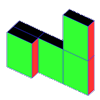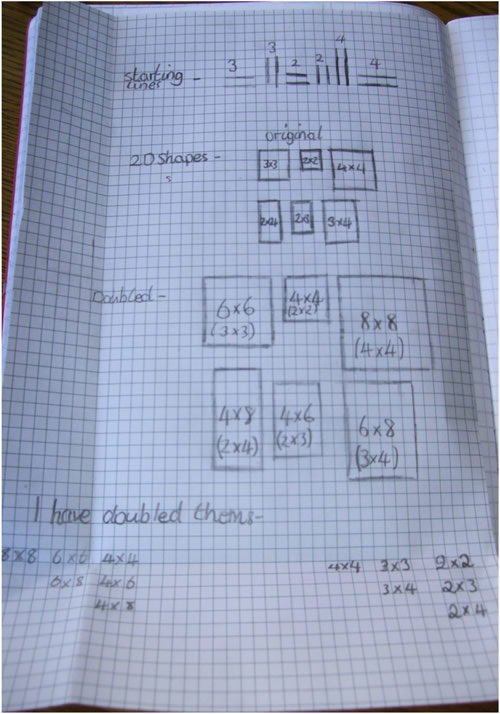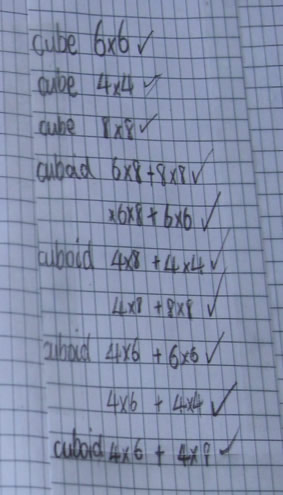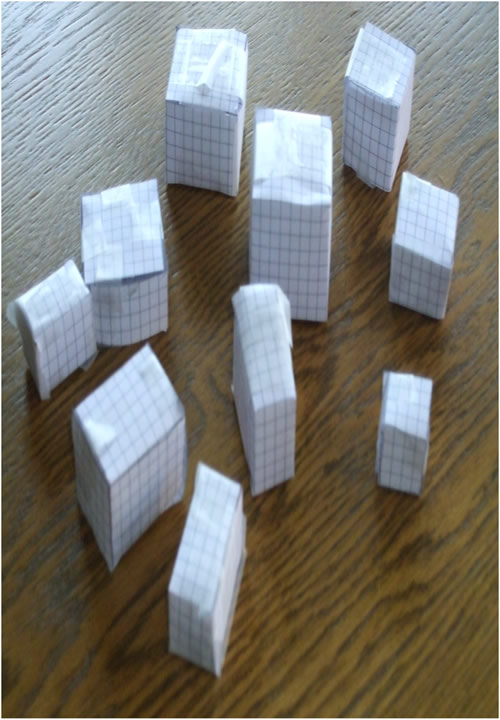#### You may also likeIf you had 36 cubes, what different cuboids could you make?### Construct-o-straws

Make a cube out of straws and have a go at this practical challenge.### Cereal Packets

How can you put five cereal packets together to make different shapes if you must put them face-to-face?

# Making Cuboids

##### Age 7 to 11Challenge Level

There are lots of answers to this problem, depending on what questions you choose to ask.

Here is some work that Lydia from St Mary's Catholic High School in Chesterfield sent to us:

There must be ten cuboids:

 $6\times6\times6$ $4\times4\times4$ $8\times8\times8$ $6\times8\times8$ $6\times8\times6$ $4\times8\times4$ $3\times3\times3$ $2\times2\times2$ $2\times4\times4$ $3\times4\times4$ $3\times4\times3$ $2\times4\times2$

 $4\times8\times8$ $4\times6\times6$ $4\times6\times4$ $4\times6\times8$ $2\times4\times4$ $2\times3\times3$ $2\times3\times2$ $2\times3\times4$

These are the only possible shapes using the three lines (edges) with $2, 3$ and $4$ units of length.

To make these cuboids I had to double the length of each edge so I ended up with $4, 6$ and $8$ units of length, this made it easier to construct them.

The workings out are on the pictures below.Thank you, Lydia.  You seem to have worked hard at this challenge.